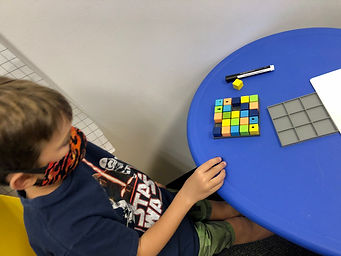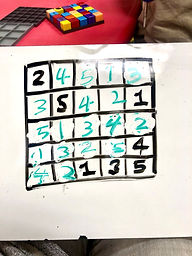Ms. AJ

Target 1​

Lesson Type:

Continuation

Critical Thinking

:

Logical Reasoning

Use reasoning to solve logic puzzles.

1:

Use the given information in a problem to identify the correct answer.

2:

Use the given information to determine which is not the correct answer.

3:

Think outside of the box to solve problems.

4:

Follow given rules to solve problems.

4th

Vocabulary:

Sudoku, Column, Row, Strategy, Process of Elimination

Activities:

Students used color blocks to solve a variety of Sudoku puzzles on both 3 x 3 and 5 x 5 grids.

Students solved Sudoku puzzles with numbers on 5 x 5 grids.Home Exploration

Guiding Questions:Absent Students:

Target 2

:

1:

Use understandings of arithmetic to solve problems (where one part of the equation is given and the answer).

2:

Understand the inverse relationship between addition and subtraction.

3:

Understand the inverse relationship between multiplication and division.

6th

Vocabulary:

Missing Numbers, Variables, Symbols, Solve For

Activities:

Students learned about variables, and solved for various symbols given in equations as "missing numbers".Home Exploration

Guiding Questions:Target 3

:

Vocabulary:

Activities:Home Exploration